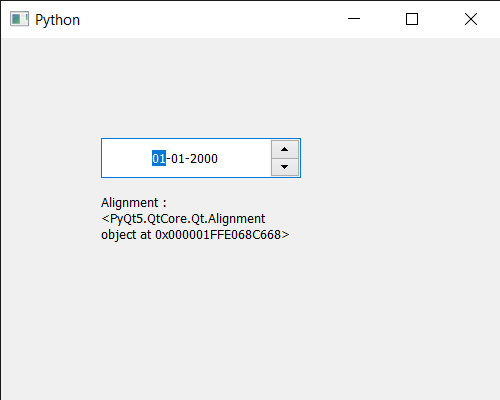Related Articles
PyQt5 QDateEdit – Getting Date Alignment
• Last Updated : 07 Jul, 2020

In this article we will see how we can get the date alignment of the QDateEdit. By default, the text is aligned on the left hand side although by changing the alignment we can make the date appear in the center with the help of `setAlignment` method. Changing alignment does not effect the functionality of the date edit.

In order to do this we use `alignment` method with the QDateEdit object

Syntax : date.alignment()

Argument : It takes no argument

Return : It returns Alignment flag object

Below is the implementation

 `# importing libraries``from` `PyQt5.QtWidgets ``import` `*` `from` `PyQt5 ``import` `QtCore, QtGui``from` `PyQt5.QtGui ``import` `*` `from` `PyQt5.QtCore ``import` `*` `import` `sys`` ` ` ` `class` `Window(QMainWindow):`` ` `    ``def` `__init__(``self``):``        ``super``().__init__()`` ` `        ``# setting title``        ``self``.setWindowTitle(``"Python "``)`` ` `        ``# setting geometry``        ``self``.setGeometry(``100``, ``100``, ``500``, ``400``)`` ` `        ``# calling method``        ``self``.UiComponents()`` ` `        ``# showing all the widgets``        ``self``.show()`` ` `    ``# method for components``    ``def` `UiComponents(``self``):`` ` `        ``# creating a QDateEdit widget``        ``date ``=` `QDateEdit(``self``)`` ` `        ``# setting geometry of the date edit``        ``date.setGeometry(``100``, ``100``, ``200``, ``40``)`` ` `        ``# alignment``        ``a_flag ``=` `Qt.AlignCenter`` ` `        ``# setting alignment of date``        ``date.setAlignment(a_flag)`` ` `        ``# creating a label``        ``label ``=` `QLabel(``"GeeksforGeeks"``, ``self``)`` ` `        ``# setting geometry``        ``label.setGeometry(``100``, ``150``, ``200``, ``60``)`` ` `        ``# making label multiline``        ``label.setWordWrap(``True``)`` ` `        ``# getting alignment``        ``value ``=` `date.alignment()`` ` `        ``# setting text to the label``        ``label.setText(``"Alignment : "` `+` `str``(value))`` ` ` ` `# create pyqt5 app``App ``=` `QApplication(sys.argv)`` ` `# create the instance of our Window``window ``=` `Window()`` ` `# start the app``sys.exit(App.``exec``())`

Output :Attention geek! Strengthen your foundations with the Python Programming Foundation Course and learn the basics.

To begin with, your interview preparations Enhance your Data Structures concepts with the Python DS Course. And to begin with your Machine Learning Journey, join the Machine Learning – Basic Level Course

My Personal Notes arrow_drop_up